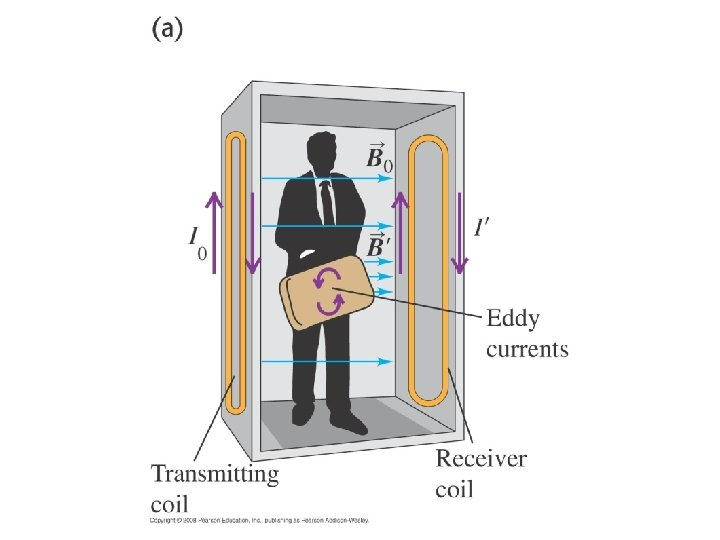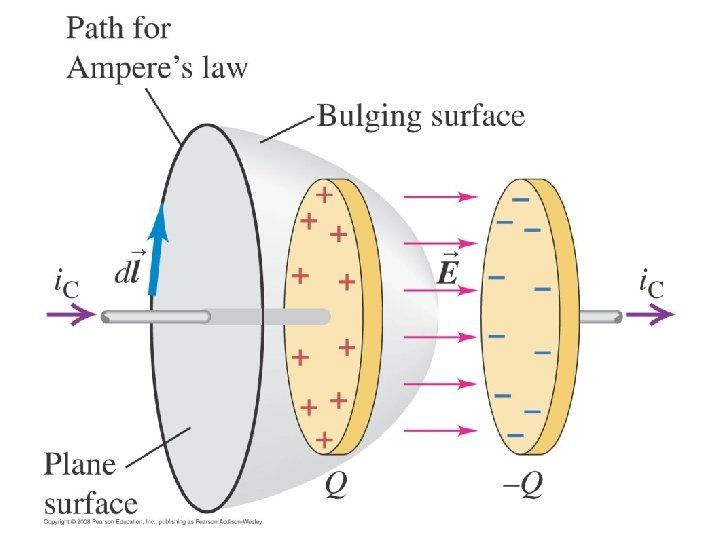# Q 29 1 A circular loop of wire

• Slides: 25Q 29. 1 A circular loop of wire is in a region of spatially uniform magnetic field. The magnetic field is directed into the plane of the figure. If the magnetic field magnitude is constant, A. the induced emf is clockwise. B. the induced emf is counterclockwise. C. the induced emf is zero. D. The answer depends on the strength of the field.A 29. 1 A circular loop of wire is in a region of spatially uniform magnetic field. The magnetic field is directed into the plane of the figure. If the magnetic field magnitude is constant, A. the induced emf is clockwise. B. the induced emf is counterclockwise. C. the induced emf is zero. D. The answer depends on the strength of the field.Q 29. 2 A circular loop of wire is in a region of spatially uniform magnetic field. The magnetic field is directed into the plane of the figure. If the magnetic field magnitude is decreasing, A. the induced emf is clockwise. B. the induced emf is counterclockwise. C. the induced emf is zero. D. The answer depends on the strength of the field.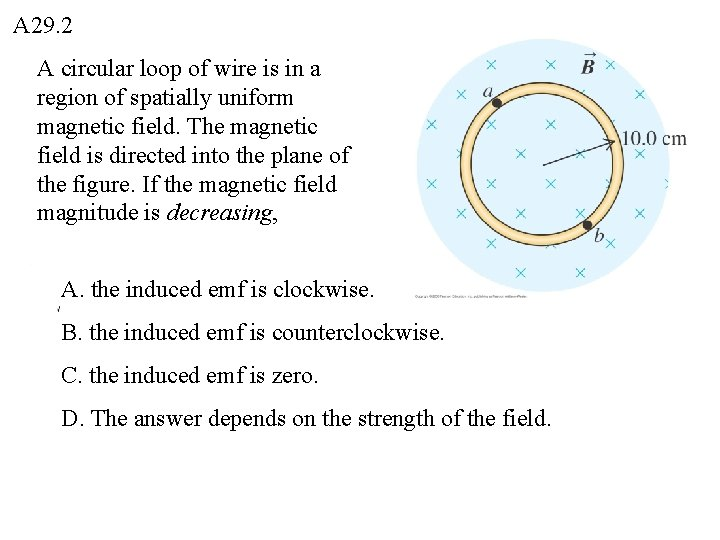A 29. 2 A circular loop of wire is in a region of spatially uniform magnetic field. The magnetic field is directed into the plane of the figure. If the magnetic field magnitude is decreasing, A. the induced emf is clockwise. B. the induced emf is counterclockwise. C. the induced emf is zero. D. The answer depends on the strength of the field.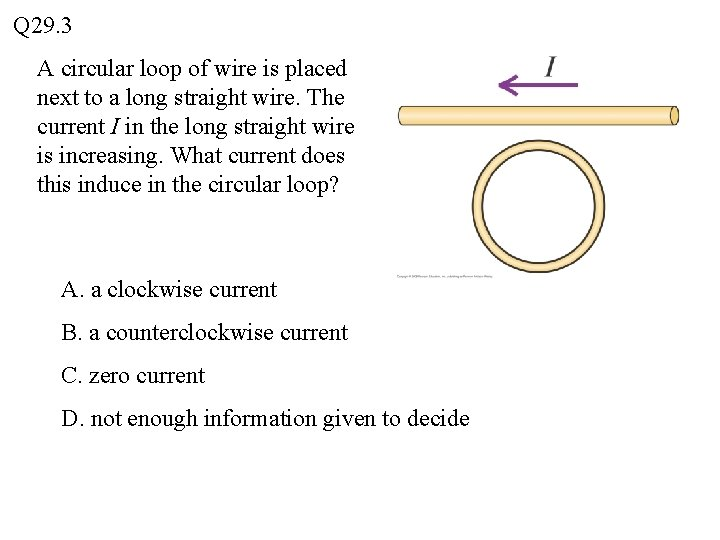Q 29. 3 A circular loop of wire is placed next to a long straight wire. The current I in the long straight wire is increasing. What current does this induce in the circular loop? A. a clockwise current B. a counterclockwise current C. zero current D. not enough information given to decide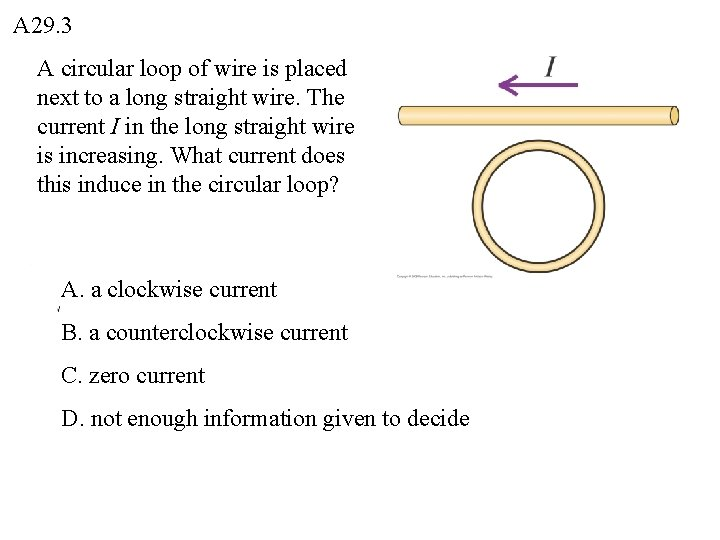A 29. 3 A circular loop of wire is placed next to a long straight wire. The current I in the long straight wire is increasing. What current does this induce in the circular loop? A. a clockwise current B. a counterclockwise current C. zero current D. not enough information given to decideQ 29. 4 A flexible loop of wire lies in a uniform magnetic field of magnitude B directed into the plane of the picture. The loop is pulled as shown, reducing its area. The induced current A. flows downward through resistor R and is proportional to B. B. flows upward through resistor R and is proportional to B. C. flows downward through resistor R and is proportional to B 2. D. flows upward through resistor R and is proportional to B 2. E. none of the above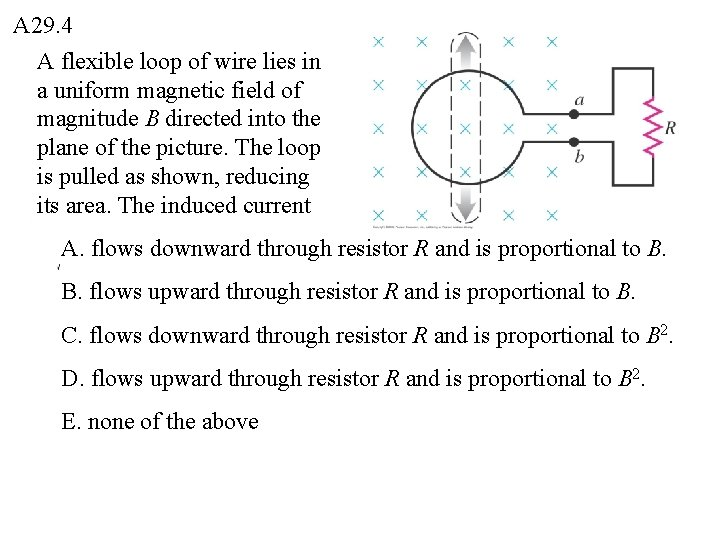A 29. 4 A flexible loop of wire lies in a uniform magnetic field of magnitude B directed into the plane of the picture. The loop is pulled as shown, reducing its area. The induced current A. flows downward through resistor R and is proportional to B. B. flows upward through resistor R and is proportional to B. C. flows downward through resistor R and is proportional to B 2. D. flows upward through resistor R and is proportional to B 2. E. none of the aboveQ 29. 5 The rectangular loop of wire is being moved to the right at constant velocity. A constant current I flows in the long straight wire in the direction shown. The current induced in the loop is A. clockwise and proportional to I. B. counterclockwise and proportional to I. C. clockwise and proportional to I 2. D. counterclockwise and proportional to I 2. E. zero.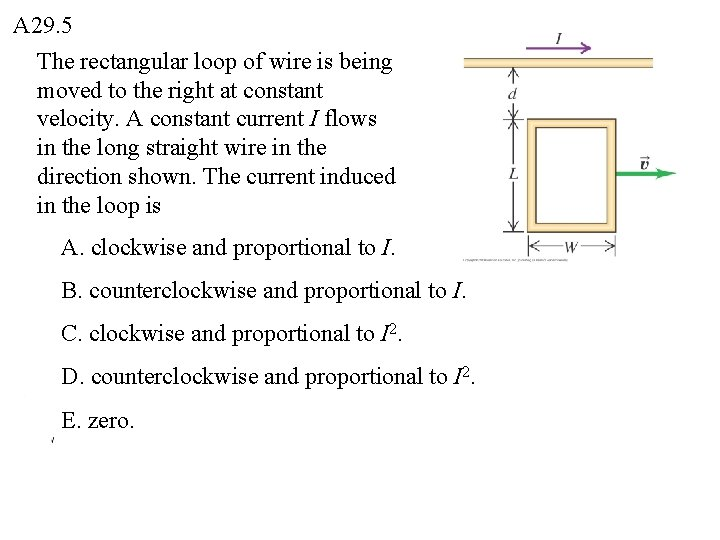A 29. 5 The rectangular loop of wire is being moved to the right at constant velocity. A constant current I flows in the long straight wire in the direction shown. The current induced in the loop is A. clockwise and proportional to I. B. counterclockwise and proportional to I. C. clockwise and proportional to I 2. D. counterclockwise and proportional to I 2. E. zero.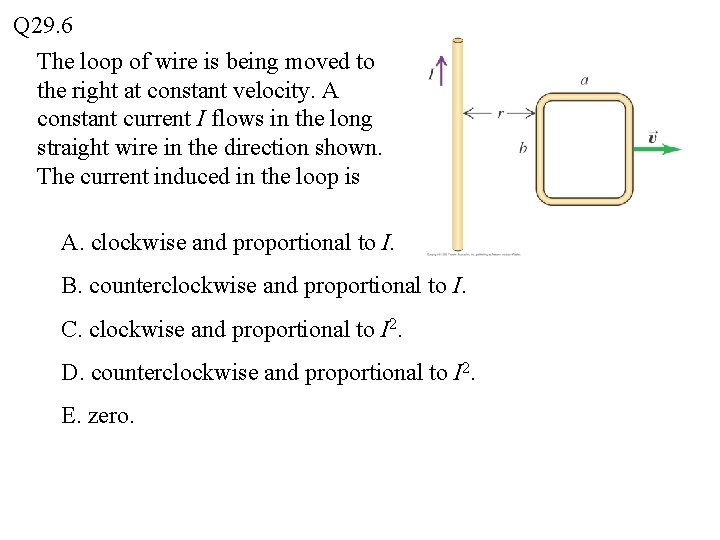Q 29. 6 The loop of wire is being moved to the right at constant velocity. A constant current I flows in the long straight wire in the direction shown. The current induced in the loop is A. clockwise and proportional to I. B. counterclockwise and proportional to I. C. clockwise and proportional to I 2. D. counterclockwise and proportional to I 2. E. zero.A 29. 6 The loop of wire is being moved to the right at constant velocity. A constant current I flows in the long straight wire in the direction shown. The current induced in the loop is A. clockwise and proportional to I. B. counterclockwise and proportional to I. C. clockwise and proportional to I 2. D. counterclockwise and proportional to I 2. E. zero.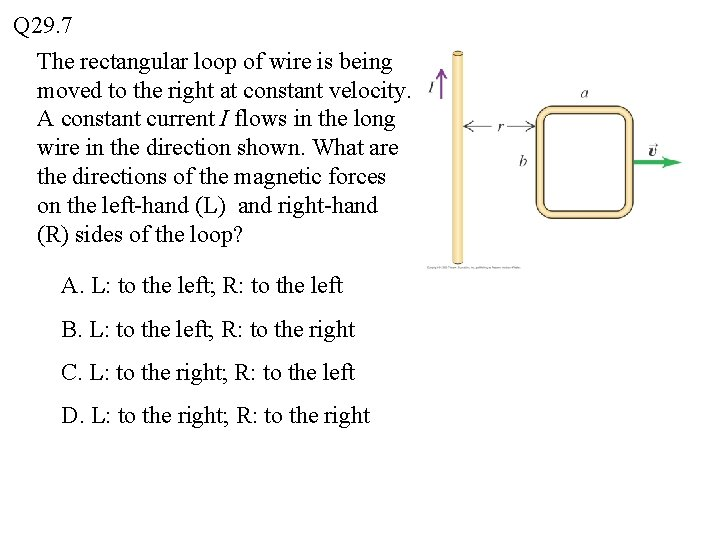Q 29. 7 The rectangular loop of wire is being moved to the right at constant velocity. A constant current I flows in the long wire in the direction shown. What are the directions of the magnetic forces on the left-hand (L) and right-hand (R) sides of the loop? A. L: to the left; R: to the left B. L: to the left; R: to the right C. L: to the right; R: to the left D. L: to the right; R: to the right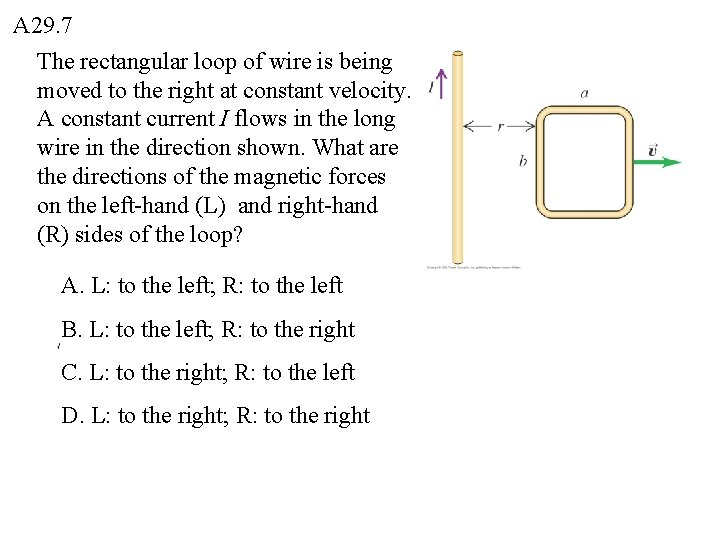A 29. 7 The rectangular loop of wire is being moved to the right at constant velocity. A constant current I flows in the long wire in the direction shown. What are the directions of the magnetic forces on the left-hand (L) and right-hand (R) sides of the loop? A. L: to the left; R: to the left B. L: to the left; R: to the right C. L: to the right; R: to the left D. L: to the right; R: to the rightQ 29. 8 The drawing shows the uniform magnetic field inside a long, straight solenoid. The field is directed into the plane of the drawing, and is increasing. What is the direction of the electric force on a positive point charge placed at point a? A. to the left B. to the right C. straight up D. straight down E. misleading question — the electric force at this point is zero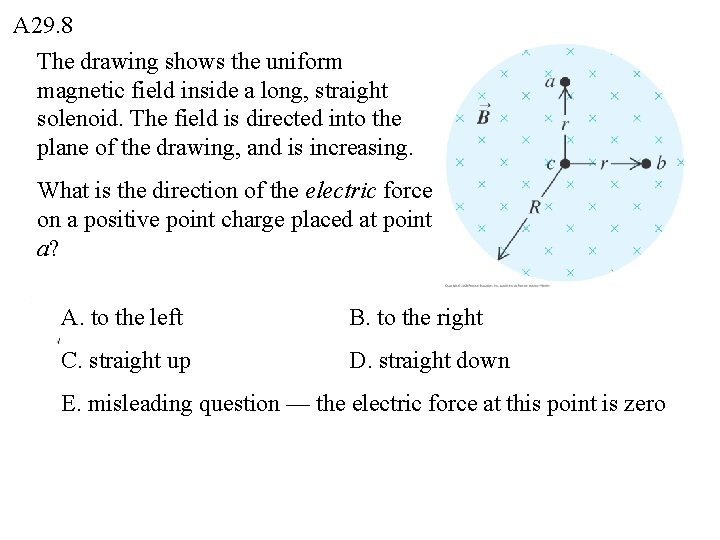A 29. 8 The drawing shows the uniform magnetic field inside a long, straight solenoid. The field is directed into the plane of the drawing, and is increasing. What is the direction of the electric force on a positive point charge placed at point a? A. to the left B. to the right C. straight up D. straight down E. misleading question — the electric force at this point is zero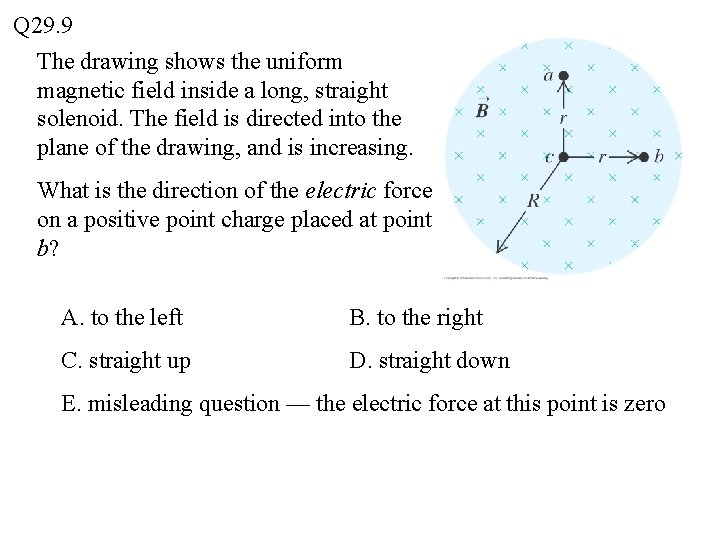Q 29. 9 The drawing shows the uniform magnetic field inside a long, straight solenoid. The field is directed into the plane of the drawing, and is increasing. What is the direction of the electric force on a positive point charge placed at point b? A. to the left B. to the right C. straight up D. straight down E. misleading question — the electric force at this point is zero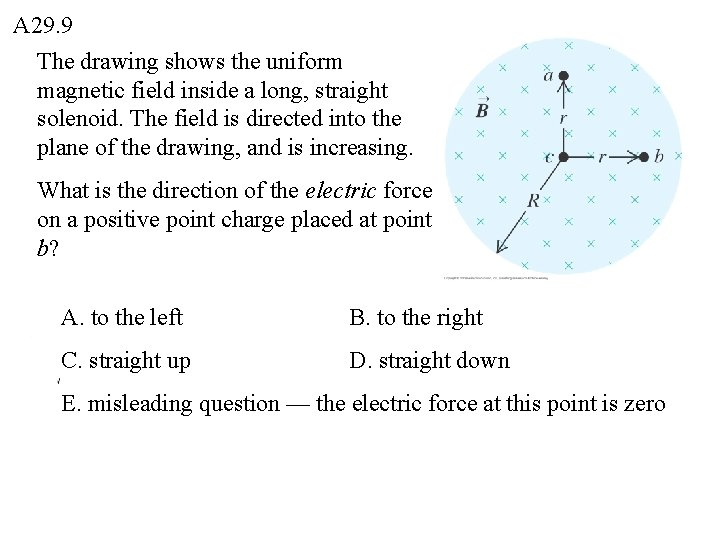A 29. 9 The drawing shows the uniform magnetic field inside a long, straight solenoid. The field is directed into the plane of the drawing, and is increasing. What is the direction of the electric force on a positive point charge placed at point b? A. to the left B. to the right C. straight up D. straight down E. misleading question — the electric force at this point is zeroQ 29. 10 The drawing shows the uniform magnetic field inside a long, straight solenoid. The field is directed into the plane of the drawing, and is increasing. What is the direction of the electric force on a positive point charge placed at point c (at the center of the solenoid)? A. to the left B. to the right C. straight up D. straight down E. misleading question — the electric force at this point is zeroA 29. 10 The drawing shows the uniform magnetic field inside a long, straight solenoid. The field is directed into the plane of the drawing, and is increasing. What is the direction of the electric force on a positive point charge placed at point c (at the center of the solenoid)? A. to the left B. to the right C. straight up D. straight down E. misleading question — the electric force at this point is zero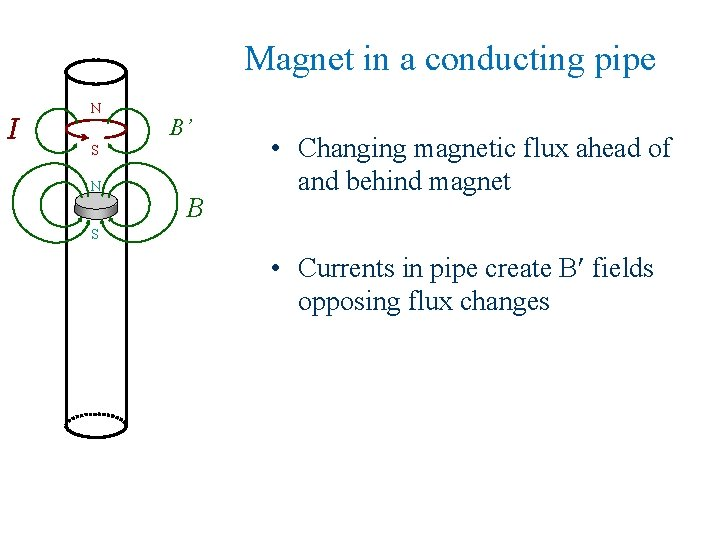Magnet in a conducting pipe I N B’ S N B • Changing magnetic flux ahead of and behind magnet S • Currents in pipe create B fields opposing flux changesThe induced current I will A) B) C) D) slow down the falling magnet speed up the falling magnet not affect it too hard to tell